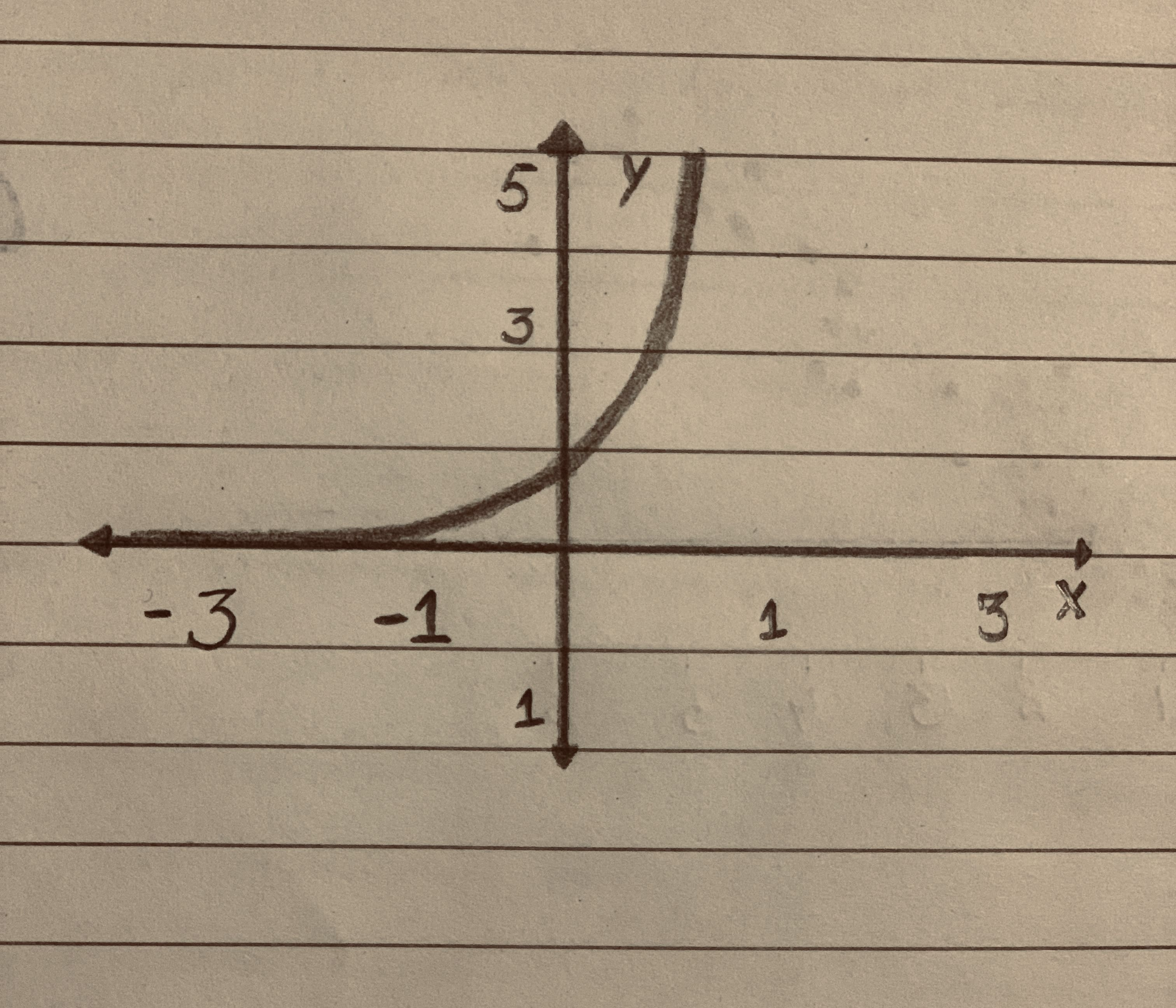UkusakazaL

2020-11-20

Determine the type of graph Increasing Linear, Decreasing Linear, Positive Quadratic, Negative Quadratic, Exponential Growth, or Exponential decay and give proof.Bertha Stark

The curve for a linear equation is always a straight line.
From the given graph, it can be seen that the given curve is not a straight line. Hence, the given graph is not increasing linear or decreasing linear.
The curve for a quadratic equation is always a parabola parallel to Y-axis.
From the given graph, it can be seen that the given curve is not a parabola. Hence, the given graph is not a graph of negative quadratic or positive quadratic function.
The curve for any exponential function passes through the point (0,1).
From the given graph, it can be seen that the given curve passes through the point (0,1). Hence, the given graph is either the graph of exponential growth or exponential decay function.
But it can be seen that the given graph is an increasing graph. Thus, the given graph is the graph for an exponential growth function.

Do you have a similar question?